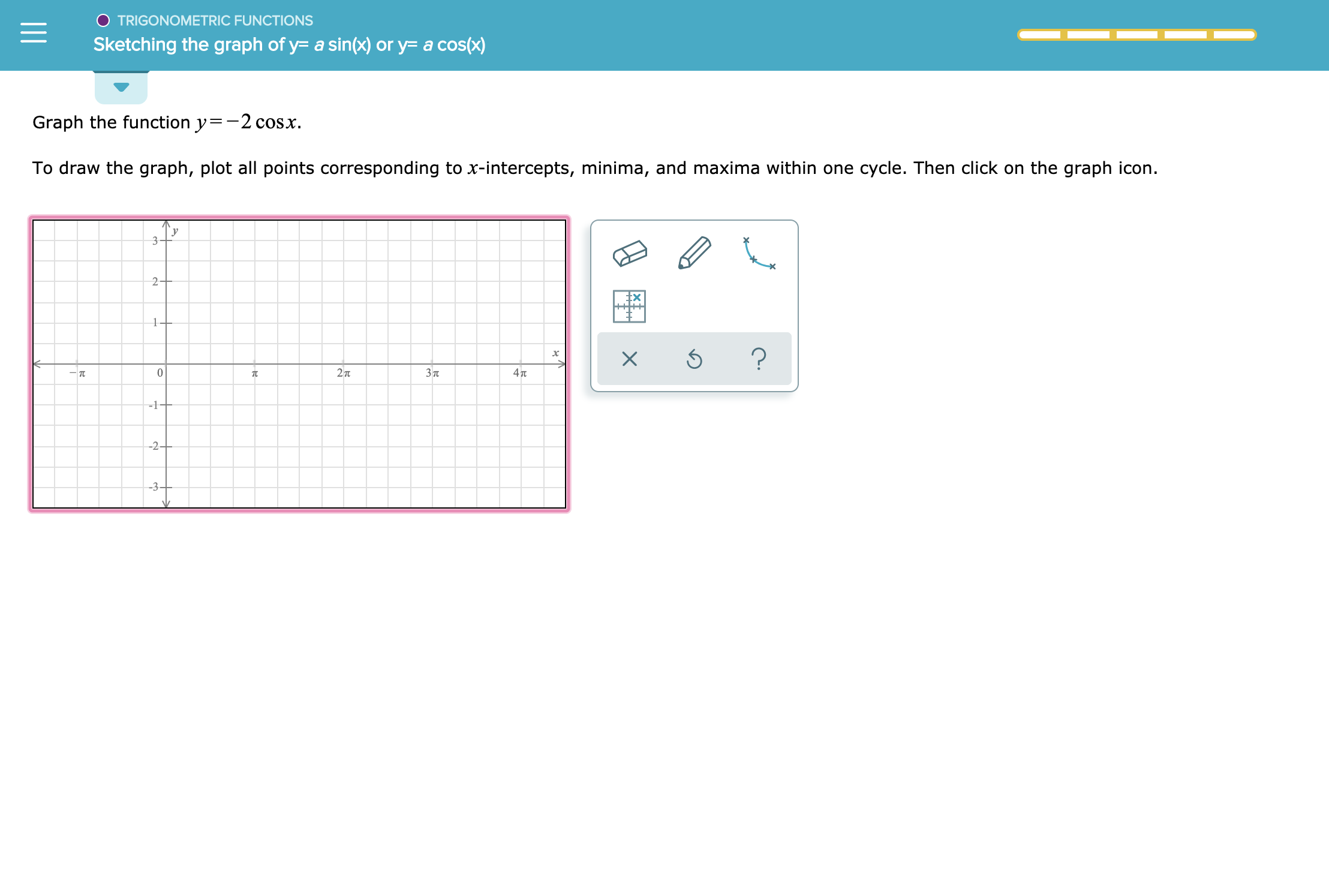# O TRIGONOMETRIC FUNCTIONSSketching the graph of y- a sin(x) or y= a cos(x)Graph the function y=-2 cosxTo draw the graph, plot all points corresponding to x-intercepts, minima, and maxima within one cycle. Then click on the graph icon.32-Ex?02 73п4TT-1+-2-3X

Question
1 views

See attachmenthelp_outlineImage TranscriptioncloseO TRIGONOMETRIC FUNCTIONS Sketching the graph of y- a sin(x) or y= a cos(x) Graph the function y=-2 cosx To draw the graph, plot all points corresponding to x-intercepts, minima, and maxima within one cycle. Then click on the graph icon. 3 2- Ex ? 0 2 7 3п 4TT -1+ -2 -3 X fullscreen
check_circle

Step 1

Consider the given...

### Want to see the full answer?

See Solution

#### Want to see this answer and more?

Solutions are written by subject experts who are available 24/7. Questions are typically answered within 1 hour.*

See Solution
*Response times may vary by subject and question.
Tagged in

### Other